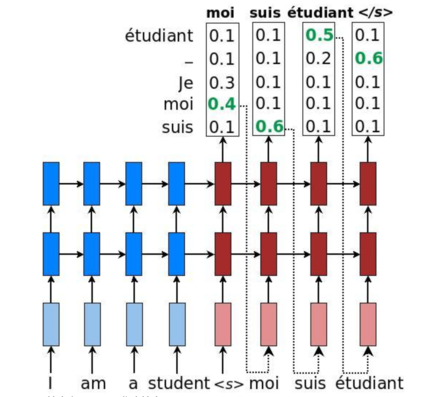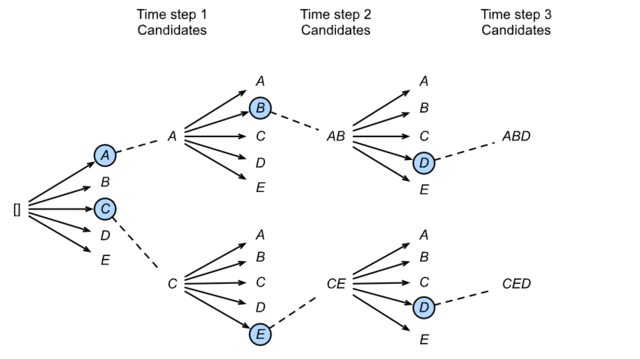# DL notes 03：机器翻译(MT)/NLP 基础知识

@[toc]

## 一、机器翻译

机器翻译（MT）：将一段文本从一种语言自动翻译为另一种语言，用神经网络解决这个问题通常称为神经机器翻译（NMT）。 主要特征：输出是单词序列而不是单个单词。 输出序列的长度可能与源序列的长度不同。

### 1.1 数据预处理和清洗

def preprocess_raw(text):
text = text.replace('\u202f', ' ').replace('\xa0', ' ')
out = ''
for i, char in enumerate(text.lower()):
if char in (',', '!', '.') and i > 0 and text[i-1] != ' ':
out += ' '
out += char
return out

### 1.2 文本预处理

#### 1.2.1 分词

# spaCy
import spacy
doc = nlp(text)
print([token.text for token in doc])

# NLTK
from nltk.tokenize import word_tokenize
from nltk import data
data.path.append('/home/kesci/input/nltk_data3784/nltk_data')
print(word_tokenize(text))

#### 1.2.2 建立字典

class Vocab(object):
def __init__(self, tokens, min_freq=0, use_special_tokens=False):
counter = count_corpus(tokens)  # :
self.token_freqs = list(counter.items())
self.idx_to_token = []
if use_special_tokens:
# padding, begin of sentence, end of sentence, unknown
self.pad, self.bos, self.eos, self.unk = (0, 1, 2, 3)
self.idx_to_token += ['<pad>', '<bos>', '<eos>', '<unk>']
else:
self.unk = 0
self.idx_to_token += ['<unk>']
self.idx_to_token += [token for token, freq in self.token_freqs
if freq >= min_freq and token not in self.idx_to_token]
self.token_to_idx = dict()
for idx, token in enumerate(self.idx_to_token):
self.token_to_idx[token] = idx

def __len__(self):
return len(self.idx_to_token)

def __getitem__(self, tokens):
if not isinstance(tokens, (list, tuple)):
return self.token_to_idx.get(tokens, self.unk)
return [self.__getitem__(token) for token in tokens]

def to_tokens(self, indices):
if not isinstance(indices, (list, tuple)):
return self.idx_to_token[indices]
return [self.idx_to_token[index] for index in indices]

def count_corpus(sentences):
tokens = [tk for st in sentences for tk in st]
return collections.Counter(tokens)  # 返回一个字典，记录每个词的出现次数

 token_freqs = sorted(counter.items(), key=lambda x:x)
token_freqs.sort(key=lambda x:x, reverse=True)

### 1.4 时序数据的采样

• $X$：“想要有直升”，$Y$：“要有直升机”
• $X$：“要有直升机”，$Y$：“有直升机，”
• $X$：“有直升机，”，$Y$：“直升机，想”
• $X$：“要和你飞到”，$Y$：“和你飞到宇”
• $X$：“和你飞到宇”，$Y$：“你飞到宇宙”
• $X$：“你飞到宇宙”，$Y$：“飞到宇宙去” 可以看到，如果序列的长度为$T$，时间步数为$n$，那么一共有$T-n$个合法的样本，但是这些样本有大量的重合，我们通常采用更加高效的采样方式。我们有两种方式对时序数据进行采样，分别是随机采样和相邻采样。

## 二、Encoder-Decoder

class Decoder(nn.Module):
def __init__(self, **kwargs):
super(Decoder, self).__init__(**kwargs)

def init_state(self, enc_outputs, *args):
raise NotImplementedError

def forward(self, X, state):
raise NotImplementedError

encoder-decoder 模式常用于NLP领域的对话系统、生成式任务（生成歌词）、翻译。

### Seq2Seq 模型初探

def SequenceMask(X, X_len,value=0):
maxlen = X.size(1)
mask = torch.arange(maxlen)[None, :].to(X_len.device) < X_len[:, None]
return X
class MaskedSoftmaxCELoss(nn.CrossEntropyLoss):
# pred shape: (batch_size, seq_len, vocab_size)
# label shape: (batch_size, seq_len)
# valid_length shape: (batch_size, )
def forward(self, pred, label, valid_length):
# the sample weights shape should be (batch_size, seq_len)
weights = torch.ones_like(label)
weights = SequenceMask(weights, valid_length).float()
self.reduction='none'
return (output*weights).mean(dim=1)
Seq2Seq模型的每个词的输出都是在整个词的字典空间中先确定分数最高的词，然后再组成整个句子，然而这样并没有对输出的整个句子的通顺程度做进一步的衡量，只依赖hidden state能够学习到上下文。通过大量的实验和应用，人们发现只考虑单个词的优化是不足够的。那如何找到最好的句子呢？一般的贪心搜索（greedy search）方法会在整个字典空间内选择最优解。如果一句话的长度为$N$个单词，单词的字典容量为$K$.则搜索空间为$K^N$,这种搜索方法叫做维特比算法. 集束搜索则以时间序列去进行逐步搜索，每时间步上只取top-n的候选结果，最终获得$n$个搜索出的候选结果，$n$称为beam size,过程如图所示：